# Introducing Expanded Step-by-step Math Solutions

September 26, 2012 —Comments Off

Step-by-step solutions, one of the most popular features for mathematics in Wolfram|Alpha, has just received a dramatic expansion in its functionality! With our new interface, you now have the ability to walk through all of our Step-by-step solutions at your own pace, revealing only one step at a time. Some of our programs will offer to guide you with hints when walking through solutions. And for common math problems, we can even show multiple ways to find the solutions. We are also very excited to introduce three new math content areas that now have Step-by-step solutions: solving equations, rational arithmetic, and verifying trigonometric identities. When you’re signed into Wolfram|Alpha, you can use this new feature three times a day. Or, when you upgrade to Wolfram|Alpha Pro, you can use it as many times as you like!

Let’s look at a new Step-by-step solution for an integral (one of the more popular math queries we receive). We’ll type “integrate cos^2(x)” into Wolfram|Alpha and then click the Step-by-step solution button in the top right of the results page.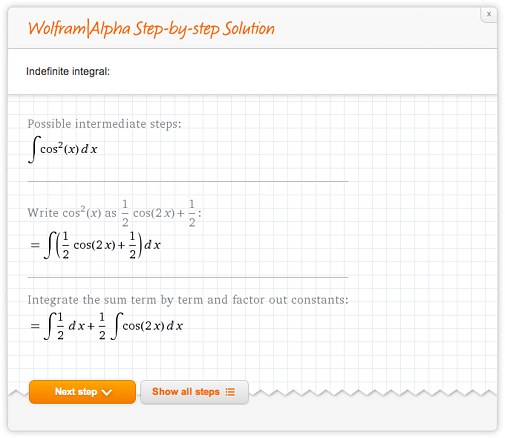To walk through the problem one step at a time, you can click the Next step button, as we have done above. Or if you’d rather see everything at once, click the Show all steps button: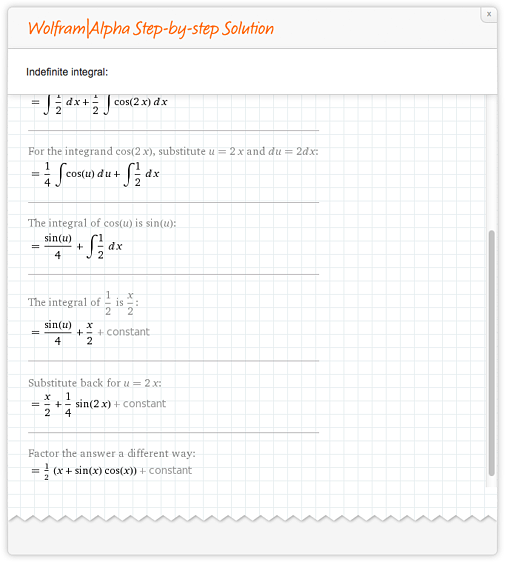Now let’s look at the input (8 * 11) / 3 + 4, which features a Step-by-step solution from one of our brand-new programs. In this walkthrough, you will have the option to use hints to help guide you through the problem: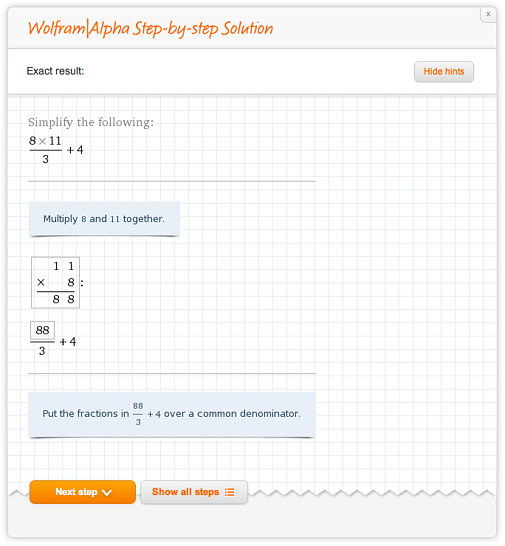As you walk through the problem, hints will give you an idea of what comes next. If you’d rather not use the hints, you can click the Hide hints button in the top right. And of course, if you’d like to see all of the steps at once, we can click “Show all steps,” as we did in our first example.

Wolfram|Alpha’s capability to show steps to solve an equation has grown tremendously over the summer! To see this, let’s start by finding the roots of a polynomial: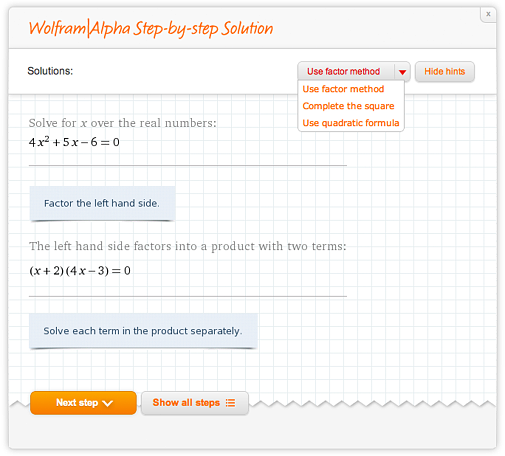The top-right corner of the Step-by-step solutions window has a drop-down menu to let us choose how to solve the problem: use the factor method, complete the square, or use the quadratic formula. Let’s try all three and compare: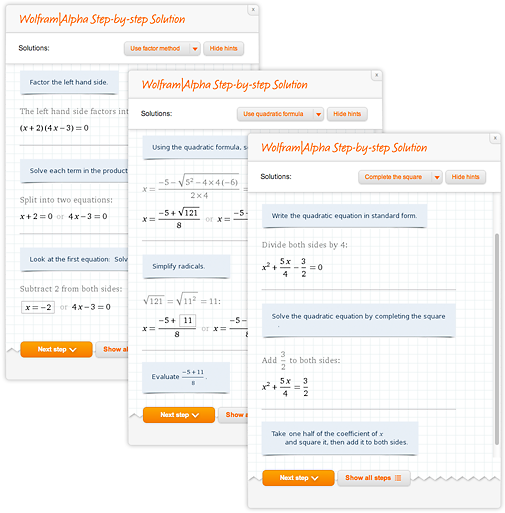Again, we see that we have the option to walk through the steps one at a time (using hints if we’d like) or to show all steps at once.

In addition to offering hints and multiple methods to solve a problem, we can now solve equations over the real numbers or over the complex numbers! Let’s see this in action by asking Wolfram|Alpha to find the roots of (e^x + 2)(x – 1). When solving over the real numbers, Wolfram|Alpha will show us that (e^x + 2)(x – 1) has only one root; over the complex numbers, Wolfram|Alpha will find the complex roots of this expression.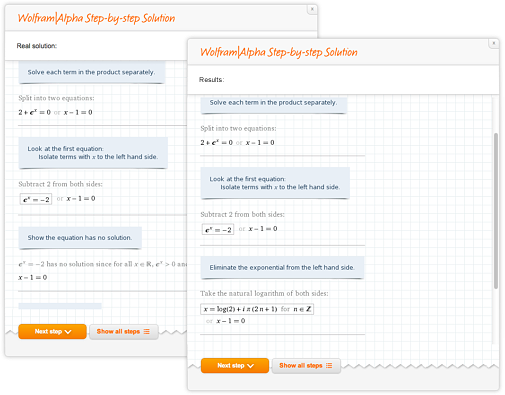To see even more of our brand-new functionality, let’s ask Wolfram|Alpha to verify a trigonometric identity. To do this, we simply type the identity we wish to prove into Wolfram|Alpha, and it will walk us through our proof one step at a time. For example, let’s try the identity (sin(x) – tan(x))(cos(x) – cot(x)) = (sin(x) – 1)(cos(x) – 1):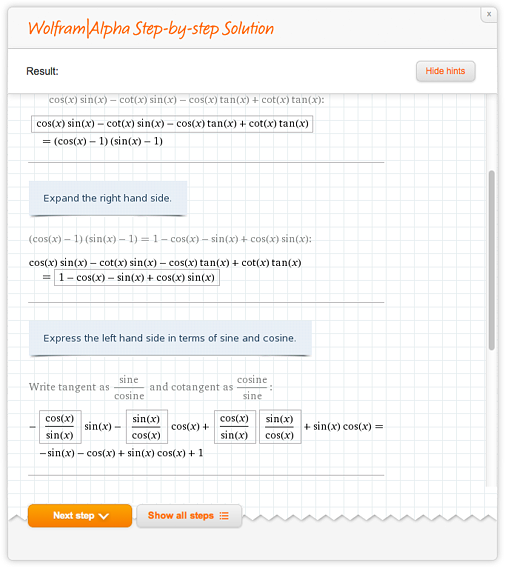Here are some more examples for you to explore the scope of Step-by-step solutions.

Arithmetic:

Equation solving:

Polynomial expansion:

Partial fraction decomposition:

Matrix row reduction:

Proving trigonometric identities:

Limits:

Derivatives:

Integrals:

Ordinary differential equations:

This gives you a brief overview of what you can do with our new Step-by-step solutions. When you’re signed into Wolfram|Alpha, you can use this new feature three times a day. Wolfram|Alpha Pro users receive unlimited access to Step-by-step solutions.

With Wolfram|Alpha’s Step-by-step Solutions feature, you can be guided—at your own pace—through a broad range of math problems, from arithmetic and equation solving all the way through integrals and ordinary differential equations. We look forward to expanding our Step-by-step solutions to more areas—please let us know if there are new solutions that you’d like to see!

I’d like to see step by step solutions of Diophantine equations, congruence equations and linear systems of congruence.

Posted by Paulo October 16, 2013 at 7:25 am

i would like to see step solution of aplication of Bisection mode to x^3=17

Posted by jose gracia December 4, 2013 at 6:22 pm

I need to know how to enter my data on the keyboard. Is the an example?

Posted by tim cundiff February 11, 2014 at 9:52 am

I would like to see Step-by-step solution for Definite Integral problems.

Posted by Jay February 23, 2014 at 1:37 pm

Yeah! stepbystep for definite integrals would be reallllyy cool. I have sometimes problems with these.. :/ and all wolfram is providing me is a straight answer 😮

Posted by Ron February 24, 2014 at 7:04 pm

I require to know the step by step to convert from Cartesian to cylindrical coordinates are derived using chain

Posted by datovi_64 March 22, 2014 at 9:34 am

How can I enter related rates problems or see examples of them?

Posted by Paul April 4, 2014 at 6:14 pm

I need know how to create a suroutne in Mathemática, I use a code several times. This way I can´t wok propoely.

Posted by Dario Magno B. Ferreira April 29, 2014 at 3:48 pm

I have several problems that remain unsolved: 1) x^4 -29x +100 = 0 solve for x but! 29 is a prime number;
if I use the quadratic I end up with a bizarre number
2) A= (x+4y)a + (2x-2y -2)b and also, B= (2x+2)a + (3x -y +1)b Suppose 3A = 2B, well, no schaum’s
says that x = 2 and y = -1 or something similar; I don’t know how to handle the small a’s & b’s
3) 6x^2 – 6y^2 +5xy +x +4y -2 = 0 Solve for y in terms of x; solve for x in terms of y
Number three is going to turn me into an alcoholic.

Posted by Teague Stapleton May 16, 2014 at 12:53 pm

Step by Step Solutions to Simulatanous Equations Please!

Posted by Jaco Delport May 19, 2014 at 6:53 am

cum se calculeaza erorile in wolfra alpha?
de exemplu eroarea unei valori (eroarea numarului 1.38629436111989
va multumesc

Posted by mihaela June 16, 2014 at 3:46 pm

2) A= (x+4y)a + (2x-2y -2)b and also, B= (2x+2)a + (3x -y +1)b Suppose 3A = 2B, well, no schaum’s?

Posted by acompanhantes são paulo July 7, 2014 at 1:33 pm

nice job sir hats off

Posted by Rakesh August 30, 2014 at 2:59 pm

The 3 free times a day is not working for me even though I have an account?

Posted by Vicky August 30, 2014 at 11:54 pm

i wanted the step by step solution for int ?^? *a^(2?-1)*exp(-(?+1/?)a^2)*BesselI[0,2*r*a/?] from a=0 to ?

thx

Posted by siavash September 18, 2014 at 5:39 pm

i wanted the step by step solution for int \beta^\nu *a^(2\nu-1)*exp(-(\beta+1/\lambda)a^2)*BesselI[0,2*r*a/\lambda] from a=0 to ?
thx

Posted by siavash September 18, 2014 at 5:42 pm

mac laurin series : f(z)= 1/ z(z^2+1) z0=i ; 1<|z-i|<2

Posted by artemis September 29, 2014 at 1:16 am

2x ? 4y = 4
4x ? 8y = 5

doesnt work… should be a simple solve no step by step, nothing..

Posted by james October 1, 2014 at 2:17 pm

i ) limit 3x + 2
x – 0 x
__________
x

ii) limit f(x), where f(x) = 6x – 8, if x 4

Posted by amymin October 1, 2014 at 8:53 pm

Hello!
Can I get Step-by-Step solutions with Wolfram’s API?
I’ve tried, but had no deal with it ;(
Thnx!

Posted by d.ilin December 16, 2014 at 5:34 am

I have several problems that remain unsolved: 1) x^4 -29x +100 = 0 solve for x but! 29 is a prime number;
if I use the quadratic I end up with a bizarre number

Posted by chácara festas July 13, 2015 at 9:53 am

I’d like to see the steps for inverting and multiplying matrices and vectors questions like finding the distance between 2 planes

Posted by Step maths October 24, 2015 at 5:32 am